•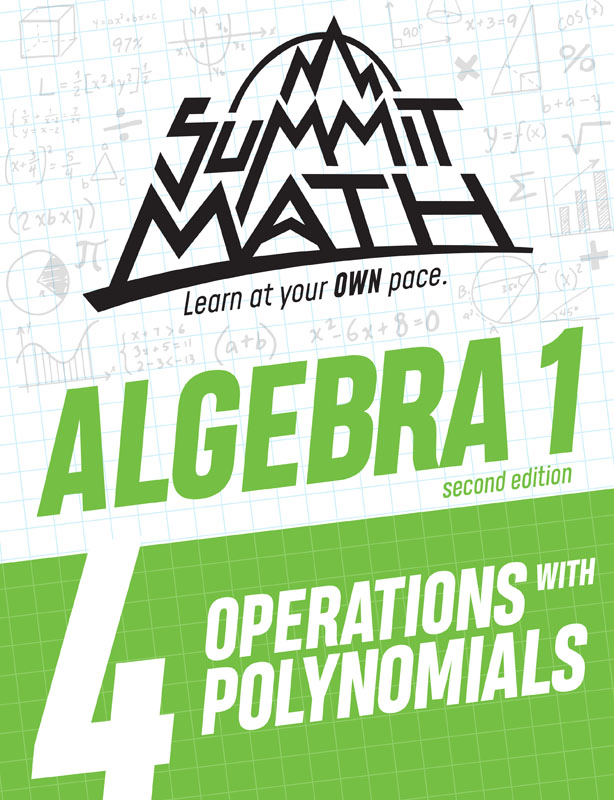•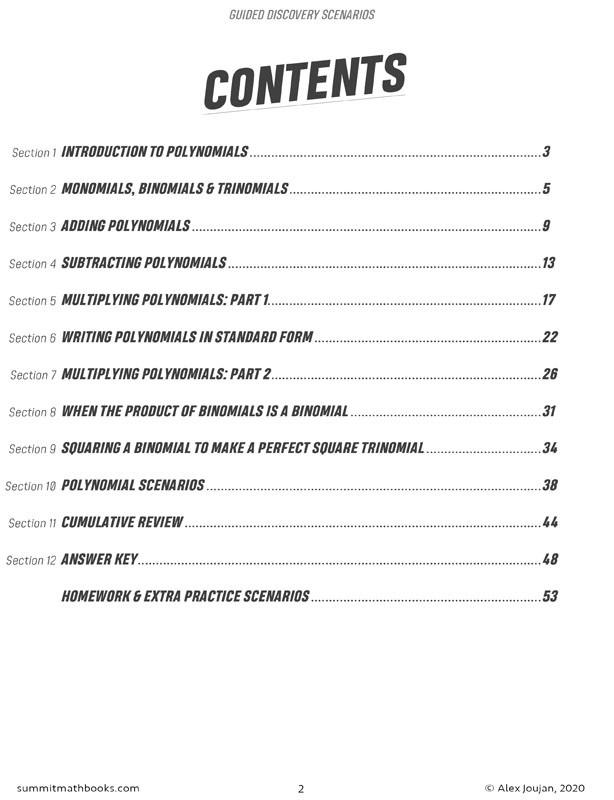•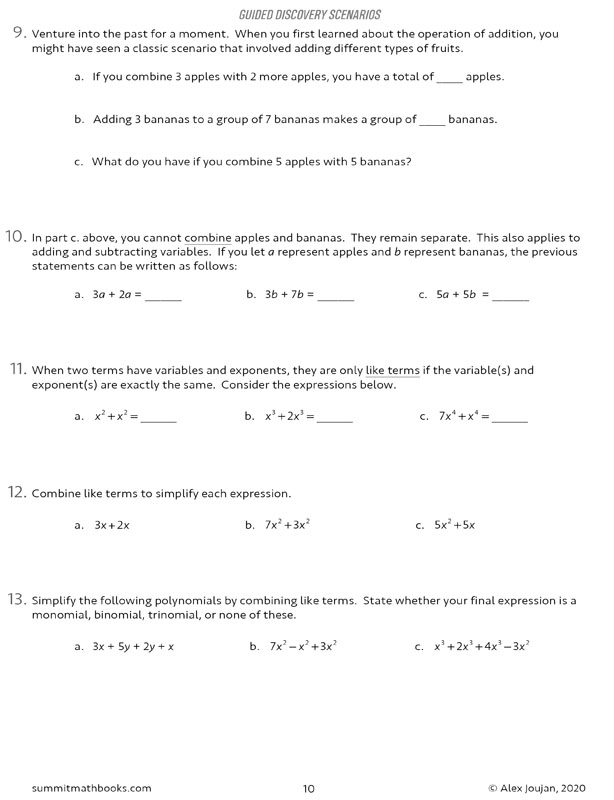•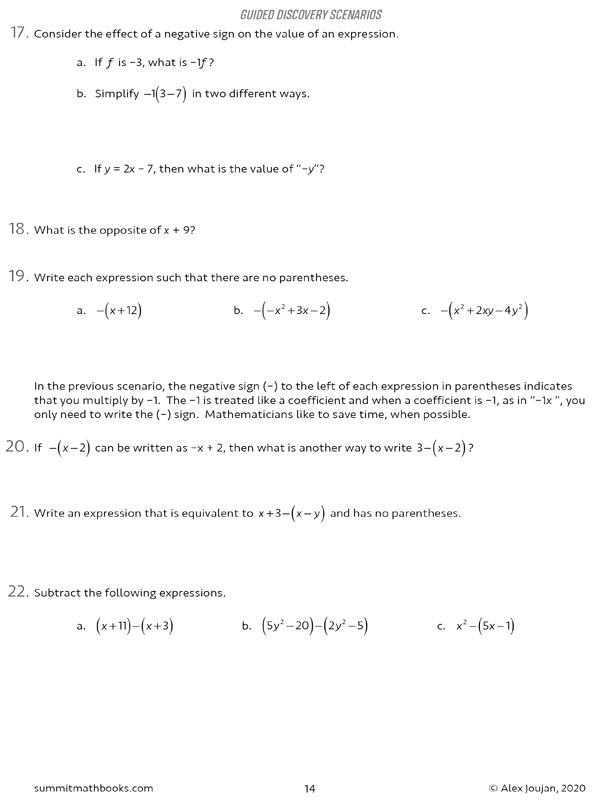•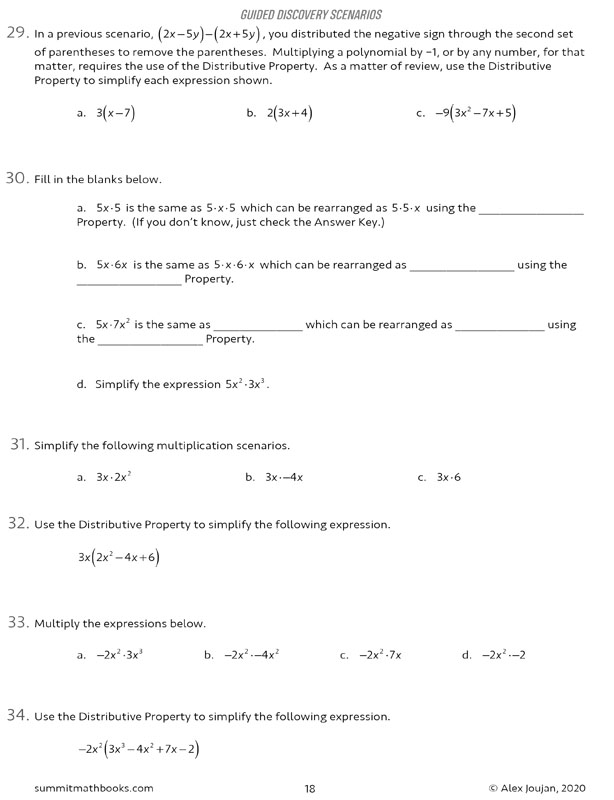•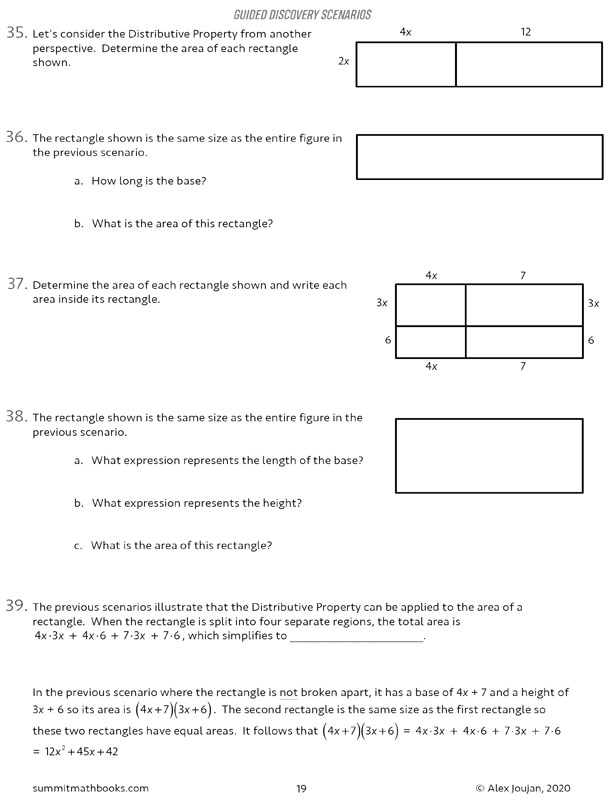# Summit Math Algebra 1 Book 4: Operations with Polynomials (2nd Edition)

# 078916

Our Price: \$12.25
Retail: \$12.50
Save: 2.00% (\$0.25)
In Stock.
Qty:
Qty:

Item #: 078916 9781712600092 6-10

#### Publisher Description:

In this book, students learn about polynomial expressions and then they use what they have learned about operations with numbers to discover how to add and subtract polynomials. Students use what they have already learned about the distributive property to discover how to multiply binomial and trinomial expressions. They also investigate special cases that occur when multiplying binomials. They explore cases where the product of two binomials is a binomial and they look for patterns that occur when a binomial is multiplied by itself. This book prepares students to learn about factoring in Algebra 1: Book 5.

Topics in this book:

• Introduction to polynomials
• Monomials, binomials, and trinomials
• Subtracting polynomials
• Multiplying polynomials: part 1
• Writing polynomials in standard form
• Multiplying polynomials: part 2
• Multiplying binomials to form another binomial
• Squaring a binomial to make a perfect square trinomial
• Polynomial scenarios
• Cumulative Review

Category Description for Summit Math Algebra:

If you are searching for an independent algebra program or a supplement to teach specific algebra concepts, Summit Math is worth your consideration. Using the guided discovery method, students make their own connections as they learn to master each scenario before moving on to the next. Each section builds on previous concepts already learned. The first half of the book incorporates the guided discovery approach, while the latter half uses extra practice sheets to improve understanding of the topics. Each section has an answer key. A placement test and pacing guide is provided for each level. The author states this is a complete program teaching typical topics in the Algebra I and II sequence while preparing students for standardized testing. If students feel they need extra instruction, a video subscription is available from the publisher. Free downloadable tests and a more detailed version of the answer key are also available at the publisher’s website.

Primary Subject
Mathematics
6
10
ISBN
9781712600092
Format
Paperback
Brand Name
Summit Math
Weight
0.65 (lbs.)
Dimensions
11.0" x 8.5" x 0.38"